## TIFR PHD CS & SS 2015

 Question 1
Consider a 6-sided die with all sides not necessarily equally likely such that probability of an even number is P({2,4,6})=1/2, probability of a multiple of 3 is P({3,6})=1/3, and probability of 1 is P({1})=1/6. Given the above conditions, choose the strongest (most stringent) condition of the following that must always hold about P({5}), the probability of 5
 A P({5})=1/6 B P({5})≥1/6 C P({5})≤1/6 D P({5})≤1/3 E None of the above
Engineering-Mathematics       Probability-and-statistics
 Question 2
Consider a circle with a circumference of one unit length. Let d < 1/6. Suppose that we independently throw two arcs, each of length d, randomly on this circumference so that each arc is uniformly distributed along the circle circumference. The arc attaches itself exactly to the circumference so that arc of length d exactly covers length d of the circumference. What can be said about the probability that the two arcs do not intersect each other?
 A It equals (1 — d) B It equals (1 — 3d) C It equals (1 — 2d) D It equals 1 E It equals (1 — d)(1 — d)
Engineering-Mathematics       Probability-and-statistics
 Question 3A Can’t be determined B 1/(1 - z) C 1/(1 + z) D 1 - z9 E None of the above
 Question 4
The Boolean function obtained by adding an inverter to each and every input of an AND gate is:
 A OR B XOR C NAND D NOR E None of the above
Digital-Logic-Design       Logic-Gates
 Question 5
What is logically equivalent to “If Kareena and Parineeti go to the shopping mall then it is raining”:
 A If Kareena and Parineeti do not go to the shopping mall then it is not raining. B If Kareena and Parineeti do not go to the shopping mall then it is raining. C If it is raining then Kareena and Parineeti go to the shopping mall. D If it is not raining then Kareena and Parineeti do not go to the shopping mall. E None of the above
Engineering-Mathematics       Propositional-Logic
 Question 6
Ram has a fair coin, i.e., a toss of the coin results in either head or tail and each event happens with probability exactly half (1/2). He repeatedly tosses the coin until he gets heads in two consecutive tosses. The expected number of coin tosses that Ram does is
 A 2 B 4 C 6 D 8 E None of the above
Engineering-Mathematics       Probability-and-statistics
 Question 7
A 1 X 1 chessboard has one (1) square, a 2 X 2 chessboards has five (5) squares. Continuing along this fashion, what is the number of squares on the (regular) 8 X 8 chessboard?
 A 64 B 65 C 204 D 144 E 256
Aptitude       Numerical
 Question 8
There is a set of 2n people: n male and n female. A good party is one with equal number of males and females (including the one where none are invited). The total number of good parties is
 A 2n B n2 CDE none of the above
Engineering-Mathematics       Combinatorics
 Question 9
Consider a square of side length 2. We throw five points into the square. Consider the following statements:
(i) There will always be three points that lie on a straight line.
(ii) There will always be a line connecting a pair of points such that two points lie on one side of the line and one point on the other.
(iii) There will always be a pair of points which are at distance at most √2 from each other.
Which of the above is true:
 A (i) only B (ii) only C (iii) only D (ii) and (iii) E None of the above
Aptitude       Numerical
 Question 10A a B b C c D d E e
Engineering-Mathematics       Calculus
 Question 11
Suppose that f(x) is a continuous function such that 0.4 ≤ f(x) ≤ 0.6 for 0 ≤ x ≤ 1. Which of the following is always true?
 A f(0.5) = 0.5 B There exists x between 0 and 1 such that f(x) = 0.8x C There exists x between 0 and 0.5 such that f(x) = x. D f(0.5) > 0.5. E None of the above statements are always true.
Engineering-Mathematics       Calculus
 Question 12
Consider two independent and identically distributed random variables X and Y uniformly distributed in [0, 1]. For α ϵ [0, 1], the probability that α max(X,Y) < XY is
 A 1/(2α) B exp(1 - α) C 1 - α D (1 - α)2 E 1 - α2
Engineering-Mathematics       Probability-and-statistics
 Question 13
Imagine the first quadrant of the real plane as consisting of unit squares. A typical square has 4 corners: (i, j), (i + 1, j), (i + 1,j + 1), and (i, j + 1), where (i, j) is a pair of non-negative integers. Suppose a line segment ℓ connecting (0, 0) to (90, 1100) is drawn. We say that ℓ passes through a unit square if it passes through a point in the interior of the square. How many unit squares does ℓ pass through?
 A 98,990 B 9,900 C 1,190 D 1,180 E 1,010
 Question 14A None B Two C Three D Four E Eight
Engineering-Mathematics       Linear-Algebra
 Question 15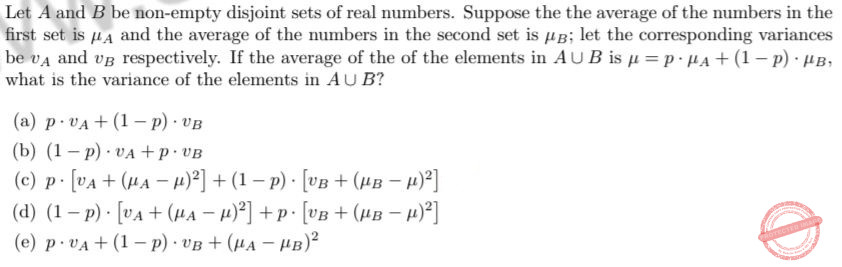A a B b C c D d E e
Engineering-Mathematics       Set-Theory
 Question 16
Consider the following recurrence relation:Which of the following statements is TRUE?
 A T(n) is O(log n). B T(n) is O(log n • log log n) but not O(log n). C T(n) is O(log3/2 n) but not O(log n • log log n). D T(n) is O(log2 n) but not O(log3/2 n). E T(n) is O(log2 n • log log n) but not O(log2 n).
Algorithms       Recurrences
 Question 17
Consider the following undirected connected graph G with weights on its edges as given in the figure below. A minimum spanning tree is a spanning tree of least weight and a maximum spanning tree is one with largest weight. A second-best minimum spanning tree is a spanning tree whose weight is the smallest among all spanning trees that are not minimum spanning trees in G.Which of the following statements is TRUE in the above graph? (Note that all the edge weights are distinct in the above graph)
 A There is more than one minimum spanning tree and similarly, there is more than one maximum spanning tree here. B There is a unique minimum spanning tree, however there is more than one maximum spanning tree here. C There is more than one minimum spanning tree, however there is a unique maximum spanning tree here. D There is more than one minimum spanning tree and similarly, there is more than one second-best minimum spanning tree here. E There is a unique minimum spanning tree, however there is more than one second-best minimum spanning tree here.
Algorithms       Minimum-Spanning-Tree
 Question 18
Consider the following code fragment in the C programming language when run on a non-negative integer n.
int f(int n)
{
if(n==0 || n==1) return 1;
else
return f(n-1) + f(n-2);
}
Assuming a typical implementation of the language, what is the running time of this algorithm and how does it compare to the optimal running time for this problem?
 A This algorithm runs in polynomial time in n but the optimal running time is exponential in n. B This algorithm runs in exponential time in n and the optimal running time is exponential in n. C This algorithm runs in exponential time in n but the optimal running time is polynomial in n. D This algorithm runs in polynomial time in n and the optimal running time is polynomial in n. E The algorithm does not terminate
Programming Programming       Functions
 Question 19
First, consider the tree on the left.On the right, the nine nodes of the tree have been assigned numbers from the set {1, 2,. .., 9} so that for every node, the numbers in its left subtree and right subtree lie in disjoint intervals (that is, all numbers in one subtree are less than all numbers in the other subtree). How many such assignments are possible? Hint: Fix a value for the root and ask what values can then appear in its left and right subtrees.
 A 29 = 512 B 24 • 32 • 5 • 9 = 6480 C 23 • 3 • 5 • 9 = 1080 D 24 = 16 E 23 • 33 = 216
Data-Structures       Binary-Trees
 Question 20A only (i) B only (ii) C only (iii) D only (iv) E (i) and (ii)
Data-Structures       Graphs-and-Tree
 Question 21
Let B consist of all binary strings beginning with a 1 whose value when converted to decimal is divisible by 7.
 A B can be recognised by a deterministic finite state automaton. B B can be recognised by a non-deterministic finite state automaton but not by a deterministic finite state automaton. C B can be recognised by a deterministic push-down automaton but not by a non-deterministic finite state automaton. D B can be recognised by a non-deterministic push-down automaton but not by a deterministic push-down automaton. E B cannot be recognised by any push down automaton, deterministic or non-deterministic
Theory-of-Computation       Push-Down-Automata-and-Finite-Automata
 Question 22
Let a, b, c be regular expressions. Which of the following identities is correct ?
 A (a + b)* = a*b* B a(b + c) = ab + c C (a + b)* = a* + b* D (ab + a)*a = a(ba + a)* E None of the above
Theory-of-Computation       Regular-Expression
 Question 23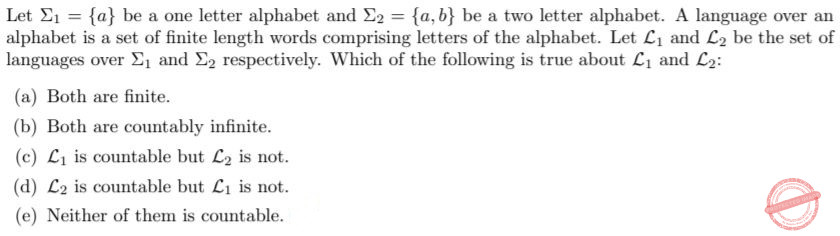A a B b C c D d E e
Theory-of-Computation       Countability
 Question 24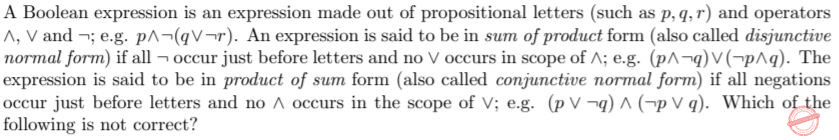A Every Boolean expression is equivalent to an expression in sum of products form. B Every Boolean expression is equivalent to an expression in product of sum form. C Every Boolean expression is equivalent to an expression without _ operator. D Every Boolean expression is equivalent to an expression without ^ operator. E Every Boolean expression is equivalent to an expression without ¬ operator.
Digital-Logic-Design       Boolean-Expression
 Question 25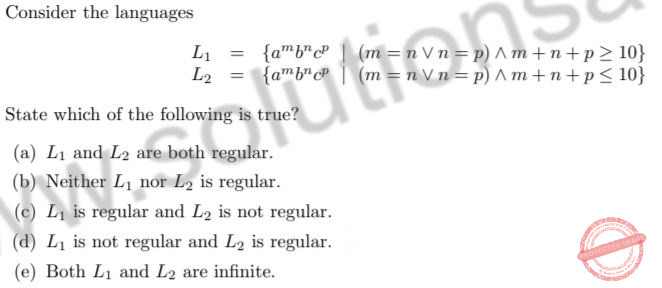A a B b C c D d E e
Theory-of-Computation       Regular-Language
 Question 26
Let Kn be the complete graph on n vertices labelled {1, 2,. .., n} with m = n(n — 1)/2 edges. What is the number of spanning trees of Kn?A a B b C c D d E e
Engineering-Mathematics       Graph-Theory
 Question 27A a B b C c D d E e
 Question 28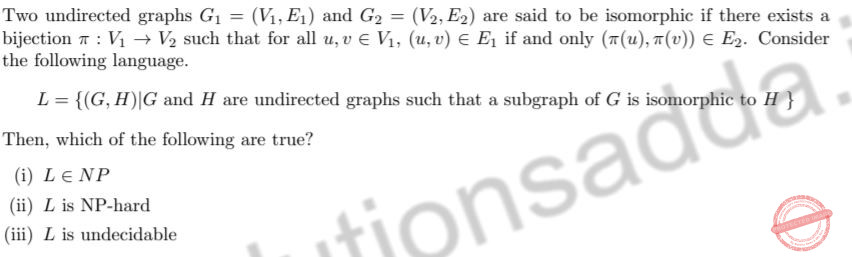A only (i) B only (ii) C only (iii) D (i) and (ii) E (ii) and (iii)
Algorithms       P-NP
 Question 29
Consider the following concurrent program (where statements separated by || within cobegin-coend are executed concurrently).
x:=1;
cobegin
x:= x+1 || x:= x+1 || x:=x+1
coend
Reading and writing of variables is atomic but evaluation of expressions is not atomic. The set of possible values of x at the end of execution of the program is
 A {4} B {2, 3, 4} C {2, 4} D {2, 3} E {2}
Programming       Operator
 Question 30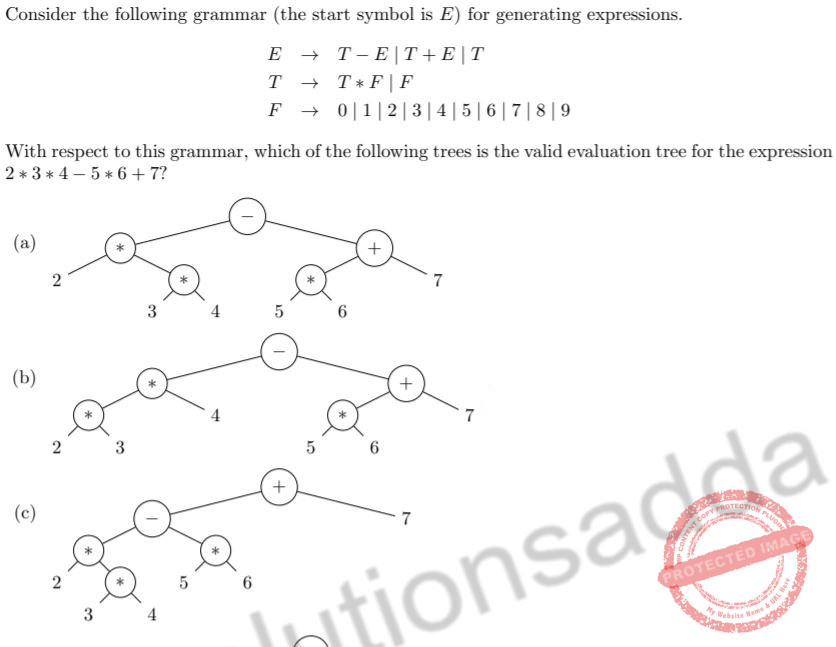A a B b C c D d E e
Compiler-Design       Grammars
 Question 31
For a time-invariant system, the impulse response completely describes the system if the system is
 A causal and non-linear B non-causal and non-linear C causal and linear D All of the above E None of the above
 Question 32A a B b C c D d E e
Engineering-Mathematics       Calculus
 Question 33
Let h(t) be the impulse response of an ideal low-pass filter with cut-o↵ frequency 5kHz. Let g[n] = h(nT), for integer n, be a sampled version of h(t) with sampling frequency 1=10kHz. The discrete time filter with g[n] as its unit impulse response is a
 A low-pass filter B high-pass filter C band-pass filter D band-stop filter E all-pass filter
 Question 34
The capacity of a certain additive white Gaussian noise channel of bandwidth 1 MHz is known to be 8 Mbps when the average transmit power constraint is 50 mW. Which of the following statements can we make about the capacity C (in Mbps) of the same channel when the average transmit power is allowed to be 100 mW?
 A C = 8 B 8 < C < 16 C C = 16 D C > 16 E There is not enough information to determine C
Computer-Networks       Noisy/Noiseless-Channel-Capacity
 Question 35A Low-pass filter B High-pass filter C Band-pass filter D Band-stop filter E All-pass filter
 Question 36A a B b C c D d E e
Engineering-Mathematics       Linear-Algebra
 Question 37A det(A) = 9 B det(A) = 18 C det(A) = 14 D det(A) = 27 E None of the above
Engineering-Mathematics       Linear-Algebra
 Question 38
Let X and Y be two independent and identically distributed random variables. Let Z = max(X, Y ) and W = min(X, Y ). Which of the following is true?
 A Z and W are independent B E(XZ) = E(YW) C E(XY ) = E(ZW) D (a), (b), and (c) E (a) and (b) only
Engineering-Mathematics       Probability-and-statistics
 Question 39
Consider a random variable X that takes integer values 1 through 10 each with equal probability. Now consider random variable
Y = min(7, max(X, 4)).
What is the variance of Y ?
 A 121/4 B 37/20 C 9/5 D 99/12 E None of the above
Engineering-Mathematics       Probability-and-statistics
 Question 40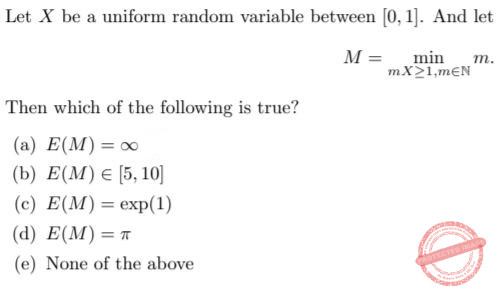A a B b C c D d E e
Engineering-Mathematics       Probability-and-statistics
 Question 41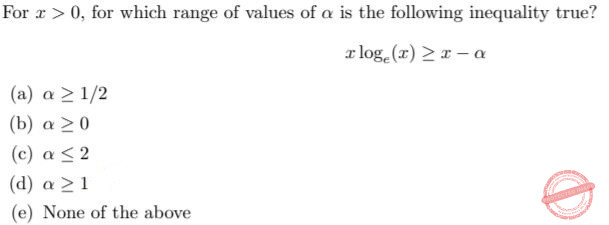A a B b C c D d E e
Engineering-Mathematics       Inequality
 Question 42
Consider the following optimization problem
max (2x + 3y)
subject to the following three constraints
x + y ≤ 5,
x + 2y ≤ 10, and
x < 3.
Let z* be the smallest number such that 2x + 3y ≤ z* for all (x, y) which satisfy the above three constraints. Which of the following is true?
 A There is no (x, y) that satisfies the above three constraints. B All (x, y) that satisfy the above three constraints have 2x + 3y strictly less than z* C There are exactly two (x, y) that satisfy the above three constraints such that 2x + 3y equals z* D There is a unique (x, y) that satisfies the above three constraints such that 2x + 3y equals z* E There are infinitely many (x, y) that satisfy the above three constraints such that 2x + 3y equals z*
Engineering-Mathematics       Inequality
 Question 43A only negative eigenvalues B only non-zero eigenvalues C only positive eigenvalues D one negative and one positive eigenvalue E None of the above
Engineering-Mathematics       Linear-Algebra
 Question 44
Consider a frog that lives on two rocks A and B and moves from one rock to the other randomly. If it is at Rock A at any time, irrespective of which rocks it occupied in the past, it jumps back to Rock A with probability 2/3 and instead jumps to Rock B with probability 1/3. Similarly, if it is at Rock B at any time, irrespective of which rocks it occupied in the past, it jumps back to Rock B with probability 2/3 and instead jumps to Rock A with probability 1/3. After the first jump, it is at Rock A. What is the limiting probability that it is at Rock A after a total of n jumps as n --> ∞?
 A 1/2 B 2/3 C 1 D The limit does not exist E None of the above
Engineering-Mathematics       Probability-and-statistics
 Question 45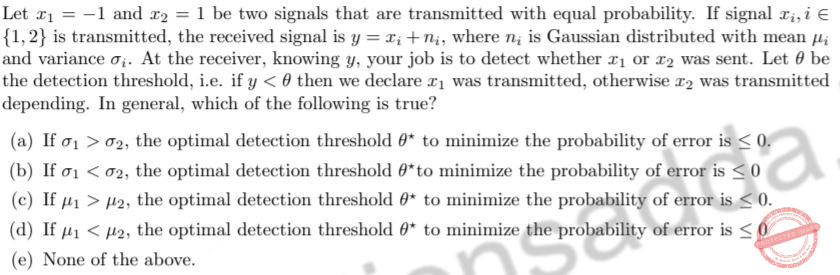A a B b C c D d E e
Engineering-Mathematics       Probability-and-statistics
There are 45 questions to complete.